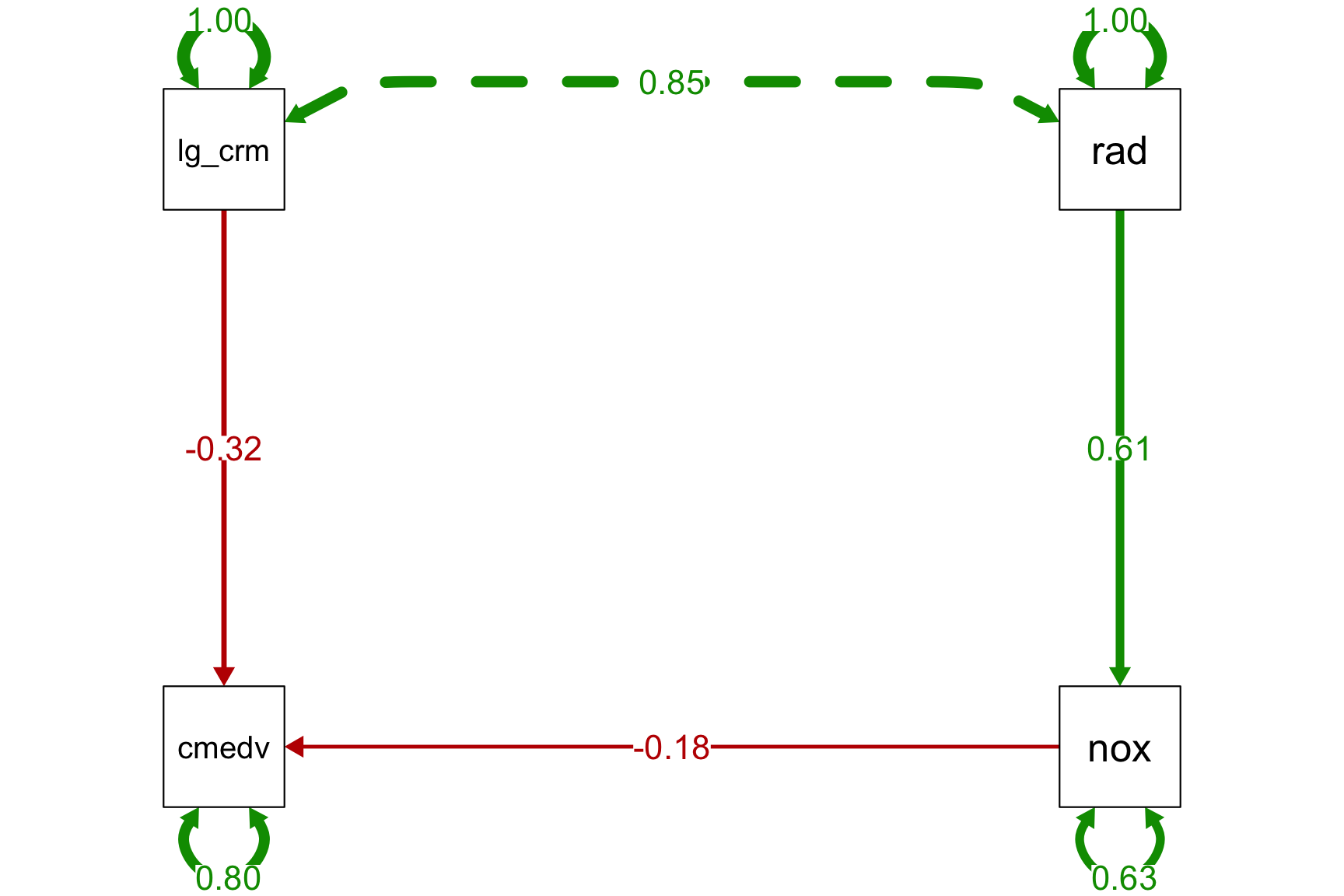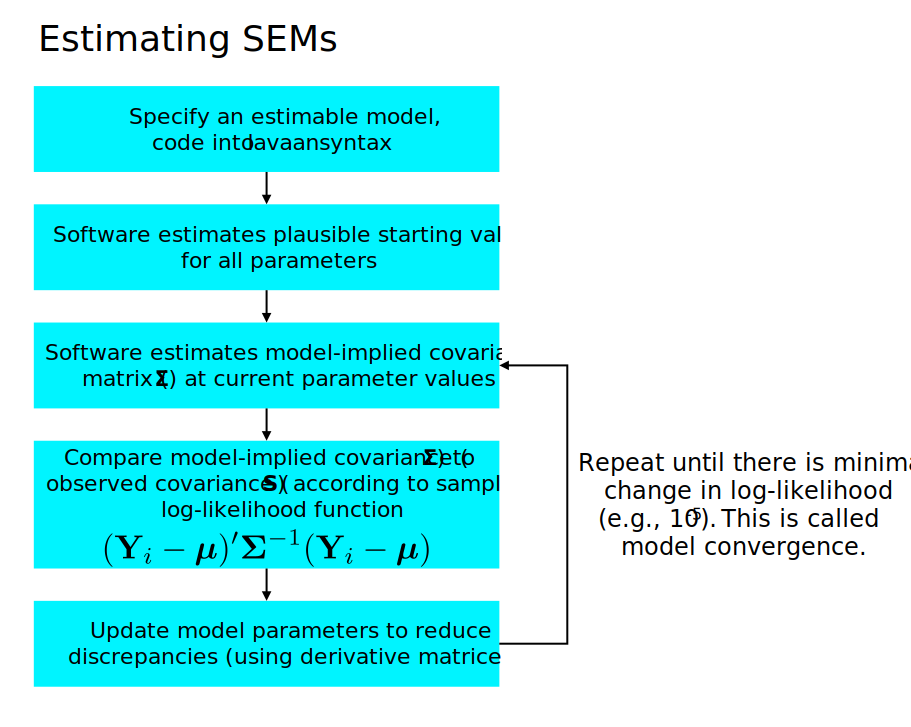If you have followed the class up to this point, you now have all of the core ingredients necessary to conduct basic SEM analyses! Now, as we move forward with using SEM to characterize a variety of datasets, there are a few odds and ends that are important to know.

# 1 Ill conditioning

Let’s return to our example from Week 1 of the class, which focused on housing prices in Boson. What if we believe that nitric oxide levels (nox) and crime predict home prices? We can add these as predictors to a path analysis, just as in standard multiple regression.

Furthermore, let’s hypothesize that the proximity of a home to large highways (rad) predicts the concentration of nitric oxides, which predicts lower home prices.

The model syntax could be specified as:

#example dataset from mlbench package with home prices in Boston by census tract
data(BostonHousing2)
BostonSmall <- BostonHousing2 %>% dplyr::select(
cmedv, #median value of home in 1000s
crim, #per capita crime by town
nox, #nitric oxide concentration
lstat, #proportion of lower status
) %>% mutate(log_crim = log2(crim))

lavaan_m2 <- '
cmedv ~ log_crim + nox #crime and nox predict lower home prices
nox ~ rad #proximity to highways predicts nox
'
mlav2 <- sem(lavaan_m2, data=BostonSmall)
## Warning in lav_data_full(data = data, group = group, cluster = cluster, :
## lavaan WARNING: some observed variances are (at least) a factor 1000 times
## larger than others; use varTable(fit) to investigate

The model looks like this (using the handy semPaths function from semPlot):

semPaths(mlav2, what='std', nCharNodes=6, sizeMan=10,
edge.label.cex=1.25, curvePivot = TRUE, fade=FALSE)Here’s the text output:

summary(mlav2)
## lavaan 0.6-3 ended normally after 33 iterations
##
##   Optimization method                           NLMINB
##   Number of free parameters                          5
##
##   Number of observations                           506
##
##   Estimator                                         ML
##   Model Fit Test Statistic                     274.360
##   Degrees of freedom                                 2
##   P-value (Chi-square)                           0.000
##
## Parameter Estimates:
##
##   Information                                 Expected
##   Information saturated (h1) model          Structured
##   Standard Errors                             Standard
##
## Regressions:
##                    Estimate  Std.Err  z-value  P(>|z|)
##   cmedv ~
##     log_crim         -0.925    0.135   -6.831    0.000
##     nox             -14.391    3.643   -3.950    0.000
##   nox ~
##     rad               0.008    0.000   17.382    0.000
##
## Variances:
##                    Estimate  Std.Err  z-value  P(>|z|)
##    .cmedv            65.499    4.118   15.906    0.000
##    .nox               0.008    0.001   15.906    0.000

A few things to note:

1. Note the warning: ‘some observed variances are (at least) a factor 1000 times larger than others.’ This is called ill conditioning.
2. Our hypotheses all seem to be supported.
3. The model chi-square is highly significant, suggesting poor global model fit.

Parameter estimation can be hampered when the variances of variables in the model differ substantially (orders of magnitude). The most common consequences are that the model does not converge or that you get warnings about problems estimating the standard errors. Here, the model did not fail in these ways, but did object to the variances. Given the above warning, let’s take a look.

varTable(mlav2)
name idx nobs type exo user mean var nlev lnam
cmedv 1 506 numeric 0 0 22.529 84.312 0
nox 3 506 numeric 0 0 0.555 0.013 0
log_crim 6 506 numeric 1 0 -1.126 9.729 0
rad 5 506 numeric 1 0 9.549 75.816 0

Looks like the scale of nox is much smaller than any other predictor, likely because it’s in parts per 10 million! We can rescale variables in this case by multiplying by a constant. This has no effect on the fit or interpretation of the model – we just have to recall what the new units represent. Also, you can always divide out the constant from the parameter estimate to recover the original units, if important.

BostonSmall <- BostonSmall %>% mutate(nox = nox*100) #not parts per 100,000 not 10 million
mlav2 <- sem(lavaan_m2, data=BostonSmall)
summary(mlav2)
## lavaan 0.6-3 ended normally after 18 iterations
##
##   Optimization method                           NLMINB
##   Number of free parameters                          5
##
##   Number of observations                           506
##
##   Estimator                                         ML
##   Model Fit Test Statistic                     274.360
##   Degrees of freedom                                 2
##   P-value (Chi-square)                           0.000
##
## Parameter Estimates:
##
##   Information                                 Expected
##   Information saturated (h1) model          Structured
##   Standard Errors                             Standard
##
## Regressions:
##                    Estimate  Std.Err  z-value  P(>|z|)
##   cmedv ~
##     log_crim         -0.925    0.135   -6.831    0.000
##     nox              -0.144    0.036   -3.950    0.000
##   nox ~
##     rad               0.814    0.047   17.382    0.000
##
## Variances:
##                    Estimate  Std.Err  z-value  P(>|z|)
##    .cmedv            65.499    4.118   15.906    0.000
##    .nox              83.910    5.275   15.906    0.000

Note that our fit statistics do not change at all (because the model converged in the first place), and our parameter estimates only change by a factor of 100, which is because we rescaled nox by multiplying the value x100.

# 2 Estimators

Estimators are algorithms that seek to fit a given model (for us, variants of SEM) to a dataset. By ‘fit a model,’ I mean that we try to identify parameter estimates that minimize the discrepancy between the observed covariance matrix, $$\boldsymbol{S}_{XX}$$ and the model-implied covariance matrix, $$\boldsymbol{\Sigma}(\hat{\boldsymbol{\theta}})$$. The algorithm searches iteratively for parameter estimates that would reduce the discrepancy, and the algorithm converges when the discrepancy cannot be further reduced by alternative parameter estimates.

Remember this:In addition to ‘ML’ – our trusty default maximum likelihood estimator – ther are many other estimators for SEMs. Here’s the synopsis from the lavaan help (http://lavaan.ugent.be/tutorial/est.html):

If all data is continuous, the default estimator in the lavaan package is maximum likelihood (estimator = “ML”). Alternative estimators available in lavaan are:

• “GLS”: generalized least squares. For complete data only.
• “WLS”: weighted least squares (sometimes called ADF estimation). For complete data only.
• “DWLS”: diagonally weighted least squares
• “ULS”: unweighted least squares

Many estimators have ‘robust’ variants, meaning that they provide robust standard errors and a scaled test statistic. For example, for the maximum likelihood estimator, lavaan provides the following robust variants:

• “MLM”: maximum likelihood estimation with robust standard errors and a Satorra-Bentler scaled test statistic. For complete data only.
• “MLMVS”: maximum likelihood estimation with robust standard errors and a mean- and variance adjusted test statistic (aka the Satterthwaite approach). For complete data only.
• “MLMV”: maximum likelihood estimation with robust standard errors and a mean- and variance adjusted test statistic (using a scale-shifted approach). For complete data only.
• “MLF”: for maximum likelihood estimation with standard errors based on the first-order derivatives, and a conventional test statistic. For both complete and incomplete data.
• “MLR”: maximum likelihood estimation with robust (Huber-White) standard errors and a scaled test statistic that is (asymptotically) equal to the Yuan-Bentler test statistic. For both complete and incomplete data.

For the DWLS and ULS estimators, lavaan also provides ‘robust’ variants: WLSM, WLSMVS, WLSMV, ULSM, ULSMVS, ULSMV. Note that for the robust WLS variants, we use the diagonal of the weight matrix for estimation, but we use the full weight matrix to correct the standard errors and to compute the test statistic.

# 3 Missing data

By default, lavaan will usually apply listwise deletion such that people missing any variable are dropped. This is usually not a great idea, both because you can lose a ton of data and because it can give a biased view of the data (for details, see Schafer and Graham, 2002, Psychological Methods). Although the details are beyond this overview, it’s usually best to use so-called full-information maximum likelhood (FIML) under an assumption that the data are missing at random (MAR) – a technical term that missingness on a given variable can be related to other variables, but not to the variable itself. Using FIML, the estimation tries to estimate all parameters based on the cases that have available data. If you want to understand this better, read Craig Enders great 2010 book, Applied Missing Data Analysis.

In lavaan, add missing='ml' to the cfa, sem, or lavaan function calls in order to request that lavaan use all available data (not just complete data) in estimating model parameters. Importantly, assuming that the data are MAR, full information estimation should improve the accuracy of parameter estimates, likely making the estimates more similar to what we would obtain if we had no missing data at all. Said more formally, FIML will produce unbiased parameter estimates and standard errors if the data are MAR or MCAR (see below).